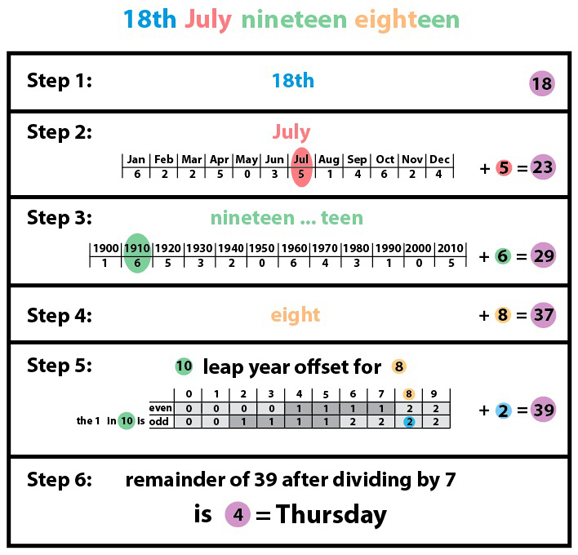# On what day of the week were you born?

March 2010

## How does it work? A detailed example

Let's see how this works using the birth date of Nelson Mandela, 18 July 1918, as an example.What the month offset table used in Step 2 really tells us is that the 30th of June in our anchor year 1950 was a Friday (which corresponds to the number 5 since Sunday=0, Monday=1, etc). Moving along by 18 days to the 18th of July takes us to the day of the week corresponding to 5+18=23. (Since 23=3×7+2, this is a Tuesday).

Step 3 takes us from 1950 to the beginning of the decade containing our date, which in this example is the year 1910. The decade offset table tells us that dates in the year 1910 are shifted along by 6 days compared to days in 1950. So we're now looking at the day corresponding to the number 5+18+6=29. (Since 29=4×7+1, this is a Monday.)

Step 4 takes account of the fact that any non-leap year shifts days along by one, so if there were no leap years between 1910 and 1918, we'd have to shift along by another 8 days. This takes us to the day corresponding to the number 5+18+6+8=37 (since 37=5×7+2, this would be a Tuesday).

Step 5 adjusts for the fact that there were in fact two leap years between 1910 and 1918, namely 1914 and 1918 itself. Each of these has one more day than an ordinary year, so this takes us to the day corresponding to 5+18+6+8+2=39. Since 39=5×7+4, this tells us that Nelson Mandela was born on a Thursday.